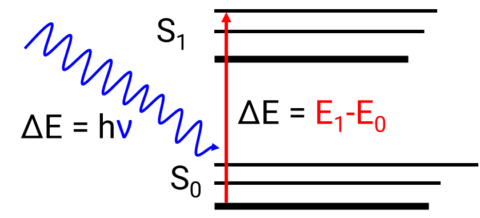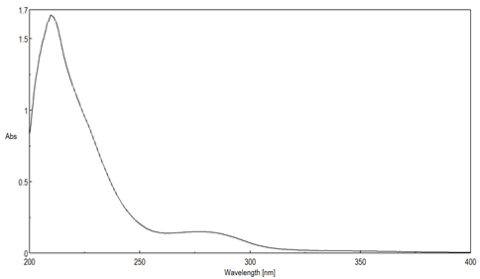# UV-Visible Spectroscopy: The Basics.

## UV-Visible Spectroscopy

UV-Visible/NIR spectroscopy (UV-Vis Spectroscopy) can be divided into ultraviolet, visible, and near-infrared regions of the spectrum, depending on the wavelengths used. since its frequency is close to the overtone frequency of many natural vibrations, weak substance-specific absorption bands can be detected. It can therefore be used for non-destructive measurements, such as determining the sugar, lipid, and protein content of foodstuffs and for identifying medicinals.

## Principle of UV-VIS Spectroscopy

UV-Visible spectroscopy exploits the wave-like nature of electrons and its interactions with electromagnetic radiation. UV-Vis spectroscopic instruments are used to identify, characterize, and quantify an extraordinary wide range of molecular compounds. It can be used for non-destructive measurements, such as determining the sugar, lipid, protein content of foodstuffs and for identifying medicinals.

When a material is irradiated with an electromagnetic wave, phenomena such as transmission, absorption, reflection, and scattering can occur. Absorption occurs when the energy of incoming light is equal to the energy difference (ΔE) between a molecule’s ground and excited states. The excitation of an electron from the ground state to an excited state is described as an electronic transition (Figure 1).Figure 1. Excitation of an electron from the ground state (S0) to the excited state (S1).

The relationship between the energy difference and wavelength is described by the Planck equation.

E=hν=hc/λ

where E is the energy required to promote an electron from the ground to an excited state, h is Planck’s constant, ν is the wavenumber, c is the speed of light, and λ is the wavelength.

Planck’s equation demonstrates that the less energy needed to excite the electrons, the longer the wavelength of the absorption band. The absorption bands are indicative of the molecular structure of the sample and will shift in wavelength and intensity depending on the molecular interaction and environmental conditions. These bands are typically broad and featureless due to the numerous molecular vibrational levels associated with the electronic energy levels.Figure 2. Example absorption spectrum. The peak around 280 nm requires less energy to promote electrons into the excited state than the peak around 215 nm.

UV-Visible/NIR spectroscopy can be divided into ultraviolet, visible, and near-infrared regions of the spectrum. The ultraviolet region is defined as 180 to 400 nm, visible between 400 and 800 nm, and the near-infrared is from 800 to 3200 nm.  Near-infrared light is generally poorly absorbed because its photon energy is insufficient to induce electronic transitions and its frequency is greater than the natural vibration frequency of most chemical bonds.  However, since the frequency in the NIR is close to the overtone frequency of many natural vibrations, weak substance-specific absorption bands can be detected.

## Beer-Lambert Law

A UV-Visible/NIR spectrophotometer measures the transmittance or the amount of light transmitted through a sample by ratioing the intensity of the incident light (I0) to the intensity of the transmitted light (I).

T= I/I0

The relationship between transmittance and absorbance is described by the following equation

abs  =   2-log⁡ I/I0 ∙100       =   2-log (%T)

Absorbance measurements are frequently used to quantify an unknown sample’s concentration by exploiting the Beer-Lambert Law which describes how light is attenuated based on the materials it passes through.  The transmittance, and therefore the absorbance, are directly proportional to a sample’s concentration, c, molar absorptivity, ε , and cuvette pathlength, l.

A= εcl

The amount of light absorbed by the sample depends on the number of molecules interacted with. The more concentrated a sample is, the more molecules are present and the higher the absorbance. Likewise, the longer the pathlength of the cell, the greater the distance that the light travels through the sample, increasing the number of molecules interacted with and therefore the absorbance. To compare the absorbances of two solutions with either different concentrations or pathlengths, there needs to be a constant variable to normalize the data on. Additionally, to determine a sample’s concentration by measuring absorbance, the cell pathlength and the strength of the electronic transition of the chromophore must be known.  This constant or the probability of the electronic transition occurring is the molar absorptivity. Since molecules have different electronic transitions of varying strengths, the molar absorptivity will vary depending on the transition being probed and is, therefore, wavelength dependent.

## UV-Visible Spectroscopy Webinar

This introductory webinar provides a review of UV-Visible theory and instrumentation basics, as well as a guide to best practices and getting good data, including information on:

• How different instrument components affect measurement results
• The difference between double beam and double monochromator instruments
• How to correctly perform a baseline measurement
• Integrating spheres and their applications